Next: Two-Dimensional Irrotational Flow in Up: Two-Dimensional Incompressible Inviscid Flow Previous: Two-Dimensional Sources and Sinks

# Two-Dimensional Vortex Filaments

Consider a vortex filament of intensity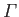that is coincident with the-axis. By symmetry, we expect the associated flow pattern to circulate isotropically around the filament. (See Figure 5.5.) In other words, we expect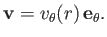(5.45)

According to Section 4.13, the circulation,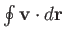, around any closed curve in the-plane is equal to the net intensity of the vortex filaments that pass through the curve. Consider a circular curve of radiusthat is concentric with the origin. It follows that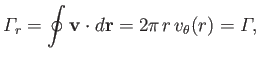(5.46)

or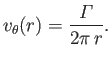(5.47)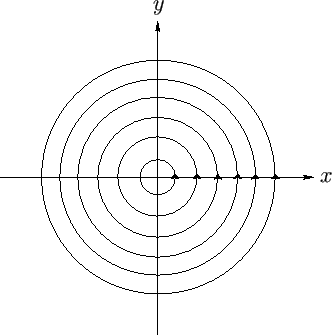According to Equations (5.13) and (5.14), the stream function associated with a vortex filament of intensitythat is coincident with the-axis is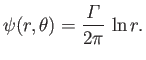(5.48)

Note that the streamlines,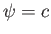, circulate around the-axis, as illustrated in Figure 5.5.

It can be seen, from Equation (5.48), that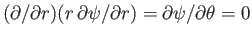. Hence, it follows from Equation (5.15) that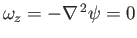. In other words, the flow pattern associated with a straight vortex filament is irrotational. This is a somewhat surprising result, because there is a net circulation of the flow around the filament, and, according to Section 4.13, non-zero circulation implies non-zero vorticity. The paradox can be resolved by supposing that the filament has a small, but finite, radius. In fact, let the filament have the finite radius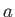, and be such that the vorticity is uniform inside this radius, and zero outside: that is,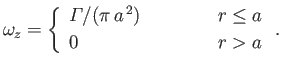(5.49)

Note that the intensity of the filament (i.e., the product of its vorticity and cross-sectional area) is still. According to Equation (5.15), and assuming that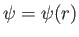,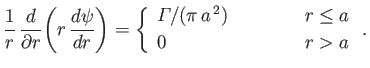(5.50)

The solution that is well behaved at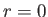, and continuous (up to its first derivative) at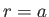, is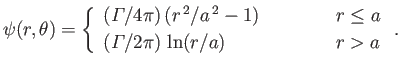(5.51)

This expression is equivalent to Equation (5.48) (apart from an unimportant additive constant) outside the filament, but differs inside. The associated circulation velocity,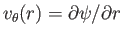, is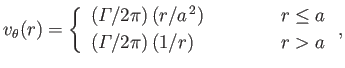(5.52)

whereas the circulation,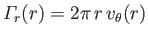, is written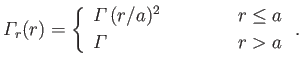(5.53)

Thus, we conclude that the flow pattern associated with a straight vortex filament is irrotational outside the filament, but has finite vorticity inside the filament. Moreover, the non-zero internal vorticity generates a constant net circulation of the flow outside the filament. In the limit in which the radius of the filament tends to zero, the vorticity within the filament tends to infinity (in such a way that the product of the vorticity and the cross-sectional area of the filament remains constant), and the region of the fluid in which the vorticity is non-zero becomes infinitesimal in extent.

Let us determined the pressure profile in the vicinity of a vortex filament of finite radius. Assuming, from symmetry, that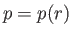, Equation (1.149), yields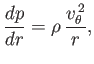(5.54)

which can be integrated to give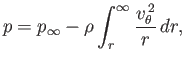(5.55)

where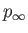is the pressure at infinity. Making use of expression (5.52), we obtain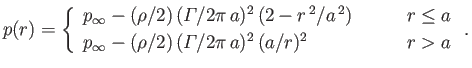(5.56)

It follows that the minimum pressure occurs at the center of the vortex (), and takes the value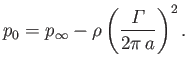(5.57)

Under normal circumstances, the pressure in a fluid must remain positive, which implies that a vortex filament of intensity, embedded in a fluid of densityand background pressure, has a minimum radius of order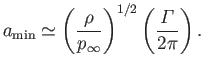(5.58)

Finally, because the flow pattern outside a straight vortex filament is irrotational, it can be derived from a velocity potential. It is easily demonstrated that the appropriate potential takes the form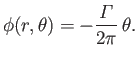(5.59)

Note that the previous potential is multivalued. However, this does not cause any particular difficulty, because the potential is continuous, and its gradient is single valued.Next: Two-Dimensional Irrotational Flow in Up: Two-Dimensional Incompressible Inviscid Flow Previous: Two-Dimensional Sources and Sinks
Richard Fitzpatrick 2016-03-31